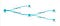State of the Art Model Deployment

Building a Decision Tree Model

```> library(XML)
> library(pmml)
> data(iris)
> library(rpart)
> irisTree <- rpart( Species~., iris )
> irisTree
n= 150
node), split, n, loss, yval, (yprob)
* denotes terminal node
1) root 150 100 setosa (0.33333333 0.33333333 0.33333333)
2) Petal.Length< 2.45 50 0 setosa (1.000000 0.0000000 0.0000000) *
3) Petal.Length>=2.45 100 50 versicolor (0.000000 0.500000 0.50000)
6) Petal.Width< 1.75 54 5 versicolor (0.0000 0.907407 0.09259) *
7) Petal.Width>=1.75 46 1 virginica (0.00000 0.021739 0.97826) *
> saveXML( pmml( irisTree ), "IrisTree.xml" )
 “IrisTree.xml”```
```from sklearn import datasets, tree
clf = tree.DecisionTreeClassifier()
clf = clf.fit(iris.data, iris.target)```
```from sklearn_pandas import DataFrameMapper
def_mapper = DataFrameMapper([(i, None) for i in iris.feature_names + ['Species']])
from sklearn2pmml import sklearn2pmml
sklearn2pmml(estimator=clf,mapper=def_mapper,pmml="IrisPyTree.xml")```
```<DataDictionary numberOfFields=”5">
<DataField name=”Species” optype=”categorical” dataType=”string”>
<Value value=”setosa”/>
<Value value=”versicolor”/>
<Value value=”virginica”/>
</DataField>
<DataField name=”Sepal.Length” optype=”continuous” dataType=”double”/>
<DataField name=”Sepal.Width” optype=”continuous” dataType=”double”/>
<DataField name=”Petal.Length” optype=”continuous” dataType=”double”/>
<DataField name=”Petal.Width” optype=”continuous” dataType=”double”/>
```<TreeModel modelName=”RPart_Model” functionName=”classification” algorithmName=”rpart”splitCharacteristic=”binarySplit” missingValueStrategy=”defaultChild” noTrueChildStrategy=”returnLastPrediction”><MiningSchema>
<MiningField name=”Species” usageType=”predicted”/>
<MiningField name=”Sepal.Length” usageType=”active”/>
<MiningField name=”Sepal.Width” usageType=”active”/>
<MiningField name=”Petal.Length” usageType=”active”/>
<MiningField name=”Petal.Width” usageType=”active”/>
</MiningSchema>```
```<Output>
<OutputField name=”Predicted_Species” optype=”categorical” dataType=”string” feature=”predictedValue”/>
<OutputField name=”Probability_setosa” optype=”continuous” dataType=”double” feature=”probability”value=”setosa”/>
<OutputField name=”Probability_versicolor” optype=”continuous” dataType=”double” feature=”probability”value=”versicolor”/>
<OutputField name=”Probability_virginica” optype=”continuous” dataType=”double” feature=”probability”value=”virginica”/>
</Output>```

Internal Elements of PMMLFigure 2. A decision tree diagram for the tree built with R.
```<Node id=”1" score=”setosa” recordCount=”150" defaultChild=”3">
<True/>
<ScoreDistribution value=”setosa” recordCount=”50" confidence=”0.333333333333333"/>
<ScoreDistribution value=”versicolor” recordCount=”50" confidence=”0.333333333333333"/>
<ScoreDistribution value=”virginica” recordCount=”50" confidence=”0.333333333333333"/>
<Node id=”2" score=”setosa” recordCount=”50">
<CompoundPredicate booleanOperator=”surrogate”>
<SimplePredicate field=”Petal.Length” operator=”lessThan” value=”2.45"/>
<SimplePredicate field=”Petal.Width” operator=”lessThan” value=”0.8"/>
<SimplePredicate field=”Sepal.Length” operator=”lessThan” value=”5.45"/>
<SimplePredicate field=”Sepal.Width” operator=”greaterOrEqual” value=”3.35"/>
</CompoundPredicate>
<ScoreDistribution value=”setosa” recordCount=”50" confidence=”1"/>
<ScoreDistribution value=”versicolor” recordCount=”0" confidence=”0"/>
<ScoreDistribution value=”virginica” recordCount=”0" confidence=”0"/>
</Node>```
```<Node id=”3" score=”versicolor” recordCount=”100" defaultChild=”7">
<CompoundPredicate booleanOperator=”surrogate”>
<SimplePredicate field=”Petal.Length” operator=”greaterOrEqual” value=”2.45"/>
<SimplePredicate field=”Petal.Width” operator=”greaterOrEqual” value=”0.8"/>
<SimplePredicate field=”Sepal.Length” operator=”greaterOrEqual” value=”5.45"/>
<SimplePredicate field=”Sepal.Width” operator=”lessThan” value=”3.35"/>
</CompoundPredicate>
<ScoreDistribution value=”setosa” recordCount=”0" confidence=”0"/>
<ScoreDistribution value=”versicolor” recordCount=”50" confidence=”0.5"/>
<ScoreDistribution value=”virginica” recordCount=”50" confidence=”0.5"/>
<Node id=”6" score=”versicolor” recordCount=”54">
<CompoundPredicate booleanOperator=”surrogate”>
<SimplePredicate field=”Petal.Width” operator=”lessThan” value=”1.75"/>
<SimplePredicate field=”Petal.Length” operator=”lessThan” value=”4.75"/>
<SimplePredicate field=”Sepal.Length” operator=”lessThan” value=”6.15"/>
<SimplePredicate field=”Sepal.Width” operator=”lessThan” value=”2.95"/>
</CompoundPredicate>
<ScoreDistribution value=”setosa” recordCount=”0" confidence=”0"/>
<ScoreDistribution value=”versicolor” recordCount=”49" confidence=”0.907407407407407"/>
<ScoreDistribution value=”virginica” recordCount=”5" confidence=”0.0925925925925926"/>
</Node>
<Node id=”7" score=”virginica” recordCount=”46">
<CompoundPredicate booleanOperator=”surrogate”>
<SimplePredicate field=”Petal.Width” operator=”greaterOrEqual” value=”1.75"/>
<SimplePredicate field=”Petal.Length” operator=”greaterOrEqual” value=”4.75"/>
<SimplePredicate field=”Sepal.Length” operator=”greaterOrEqual” value=”6.15"/>
<SimplePredicate field=”Sepal.Width” operator=”greaterOrEqual” value=”2.95"/>
</CompoundPredicate>
<ScoreDistribution value=”setosa” recordCount=”0" confidence=”0"/>
<ScoreDistribution value=”versicolor” recordCount=”1" confidence=”0.0217391304347826"/>
<ScoreDistribution value=”virginica” recordCount=”45" confidence=”0.978260869565217"/>
</Node>
</Node>```

Written by